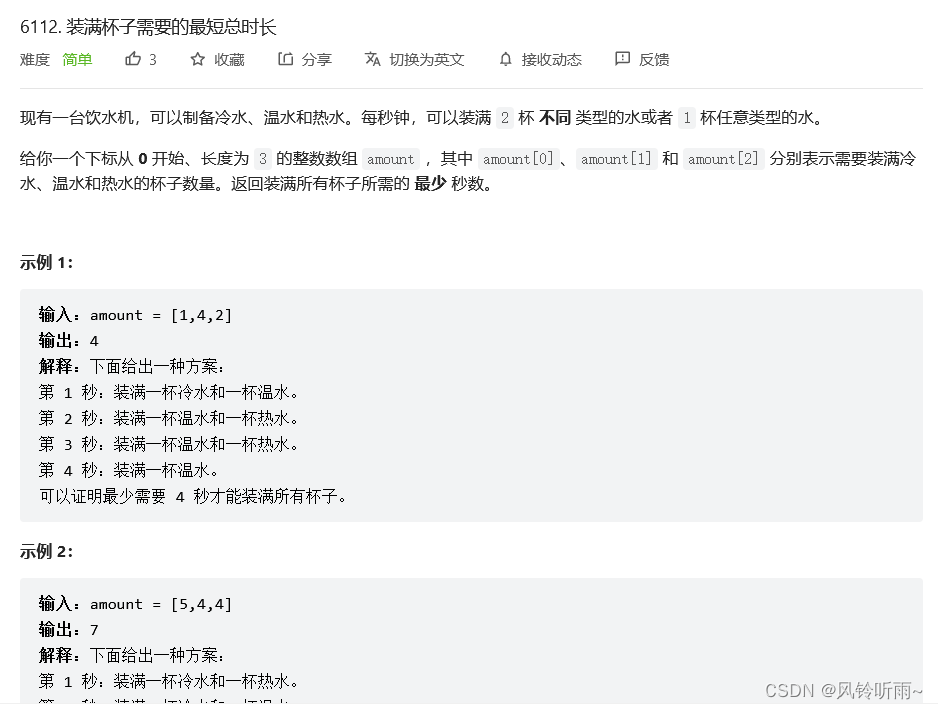﻿ Java数据结构优先队列实练_java_脚本之家
java# Java数据结构优先队列实练

## 最后一块石头的重量

### 题目描述### 代码与结果

```class Solution {
public int lastStoneWeight(int[] stones) {
PriorityQueue<Integer> pq = new PriorityQueue<Integer>((a, b) -> b - a);
for (int stone : stones) {
pq.offer(stone);
}
while (pq.size() > 1) {
int a = pq.poll();
int b = pq.poll();
if (a > b) {
pq.offer(a - b);
}
}
return pq.isEmpty() ? 0 : pq.poll();
}
}```## 装满杯子需要的最短总时长

### 题目描述### 代码与结果

```class Solution {
public int fillCups(int[] amount) {
Arrays.sort(amount);
int sum=amount+amount+amount;
if(amount+amount>=amount) sum=(sum+1)/2;
else sum=amount;
return sum;
}
}```## 移除石子的最大得分

### 题目描述### 代码与结果

```class Solution {
public int maximumScore(int a, int b, int c) {
int[] arr = new int[] { a, b, c };
Arrays.sort(arr);
a = arr;
b = arr;
c = arr;
if (a + b <= c) {
return a + b;
} else {
return (a + b + c) / 2;
}
}
}```